Time：2021-11-24

[technology blog] on continuous learning

Author: Chen Wenru

1. Summary

When learning new knowledge, people can quickly learn similar knowledge according to the previous knowledge, and can not forget the previous knowledge. While machines, or more accurately neural networks, will have some problems while learning new tasks – catastrophic forgetting. The way to solve this problem is called continuous learning. This paper focuses on some classic methods of continuous learning in recent years, in order to better understand this problem, deeply solve this problem and bring convenience to future work.
Key words: catastrophic forgetting, continuous learning

2. Introduction

When learning new knowledge, people can quickly learn similar knowledge according to the previous knowledge, and can not forget the previous knowledge. The machine, or more accurately, the neural network, will have some problems while learning new tasks – catastrophic forgetting, which means that the model learns the B of the new task and finds that the prediction is inaccurate when it goes back to predict the old task a. The problem of catastrophic forgetting is very serious, such as abnormal detection of aircraft parts. If a new part makes you forget the previous detection methods, once there is a problem, it is an incalculable disaster. So this is called catastrophic forgetting.
Therefore, in view of this phenomenon, it is necessary to propose solutions to solve catastrophic problems. We call this method continuous learning.
Continuous learning (also known as life long learning, incremental learning, etc.), which is generally called continuous learning in Chinese. Continuous learning refers to the hope that the model can quickly and accurately solve the current task based on the past a priori knowledge, but for human beings, the inherent ability is like looking for a needle in a haystack. Continuous learning must have the ability to continue previous learning, so it is also called lifelong learning, which is very vivid in the name. Continuous learning, different meta learning, different transfer learning, similar but different. The latter solves the problem of rapid learning based on experience. For example, you will 210 = 20, then you can learn 2 quickly20=40。 The focus of continuous learning is forgetting.
After reading the relevant literature on continuous learning, this paper has a general context of continuous learning, which can provide better help for the continuous learning after the project is implemented in practical application.
The main idea of continuous learning is to constrain the direction of gradient. Several methods introduced in this paper are based on gradient constraint, and the implementation effect is also good and classic.

3. Elastic weight consolidation

Elastic weight consolidation (EWC) is inspired by mammalian memory. Research has found that mammalian brain may protect previously acquired knowledge through cerebral cortical circuits, so as to avoid catastrophic forgetting. In the experiment, when a mouse needs to remember a line of skills, some synapses in the brain are strengthened (the number of dendritic spines of a single neuron increases). And even after learning other tasks in the follow-up, these increased dendritic spines can be maintained so that the relevant abilities can be retained after a few months. However, when these dendritic spines are selectively erased, the relevant skills will be forgotten. This suggests that the protection of these enhanced synapses is essential for the retention of task ability.
EWC, the main idea of this algorithm is based on the above discovery. The specific methods are briefly summarized as follows: not every node in the neural network has a great impact on the results. When learning new tasks, reducing the weight of those nodes that have too much impact on the old tasks can achieve the effect of continuous learning.

3.1 Specific method

Suppose there are two learning tasks a and B. θ A θ B is the parameter in the model of these two tasks. Task a learns first and obtains stable results. Then learn task B. in order not to let the model forget task a, it needs to be limited θ A. Make θ A is limited to a relatively low error range. Therefore, EWC can learn new tasks and θ A is treated as a secondary punishment, as shown in Figure 1. This process is like pressing the spring. For a, the spring strength should increase, so that only greater punishment can be changed θ A. It can better retain the memory of task a, while for B, the spring strength remains unchanged, which can also better remember Task B, so as to keep the memory of two tasks. The intensity of all parameters is different. For those parameters that have a great impact on task a, their intensity should be greater.
So how do you choose this strength for each parameter?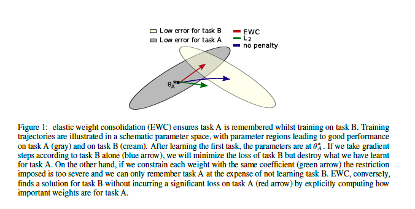3.2 Calculated strength

The author’s intention is to calculate the probability of this strength through probability. Given a data set D, through θ A priori probability, calculation θ Conditional probability of D:

• [ ]The formula is derived based on Bayesian formula: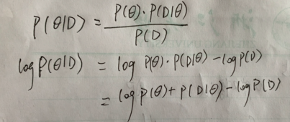LogP of the above formula（ θ The actual value of D) is, in short, the negative number of the loss value of this problem: – L（ θ)。
The above derivation is only for one task parameter. It is assumed that there are now two tasks a and B. Then this formula can be deduced again as:On the left is still the posterior probability of the parameter (given all the data), and on the right is the probability that only depends on task B。 Task a must be a posteriori probabilityAbsorption.
Since the posterior probability is difficult to obtain, the author approximates the posterior probability to a Gaussian distribution according to Laplace approximation, which is the parameter of task a θ A obtains mean and diagonal accuracy, which is given by the diagonal of Fisher information matrix (f). F has the following three important properties proved: a) f is equivalent to the approximate minimum of the second derivative of loss function; b) He can be obtained only by the first derivative of loss, so it is easy to get him; c) He guaranteed semi positive definite.Fisher information is the information about unknown parameters that can be provided by an observation θ A measure of the expected value of the amount of information. It is equivalent to a measure of the strength of the spring.
When task B is trained, a C comes. At this time, a and B can be regarded as achievement tasks, and so on.

3.2 Supervised training

A multilayer fully connected neural network is set up to train multiple supervised tasks. Shuffle the data and do small batch. Each training task has a fixed number of training times and cannot be increased.
In figure a, we can see that EWC performs very well and can remember the previous tasks, but SGD shows signs of forgetting the previous tasks in each task, and L2 regularization has catastrophic forgetting (Task B occurs when training task C).
The author took out the task SGD and compared it separately. After increasing the number of tasks, the memory decreased linearly, as shown in Figure B.
Figure C shows the effect of task similarity on Fisher matrix overlap.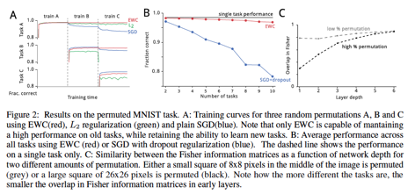4. Other relevant practices

4.1 LWF

LWF’s name is learning without forgetting. Its main idea is to deal with the problem of catastrophic forgetting through knowledge distillation.
As shown in the figure, this is the case for the normal training model. Use[ θ] () s represents the model parameters of the previous feature extraction, with θ O represents the parameters of the layer used for classification.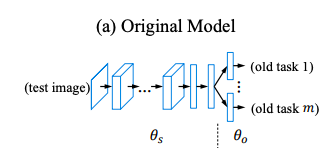The article first lists the existing schemes: the following three, as well as its own LWF.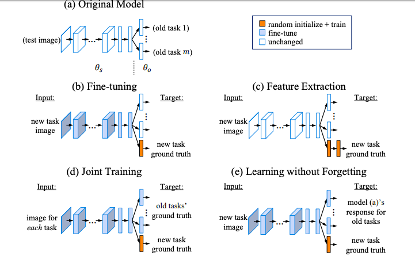Fine tuning and feature extraction are actually applicable to similar tasks, that is, the data sets are basically similar. Joint training must use the previous old data set, which is not allowed under some conditions. For example, the data needs privacy protection, and the data is too large to be saved at the same time.
So how to achieve it?
The first is pre training. Let the new ones first θ N convergence, and then joint learning by knowledge distillation.This is the loss of the new task, that is, the normal cross entropy loss (MSE). Then knowledge distillation is done by adding the loss of the original model, that is: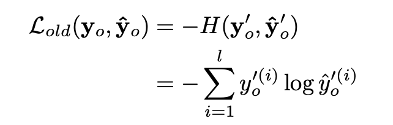This refers to the label generated by the current model and the label generated by the original model. This is an improved loss function for cross entropy loss. Of which: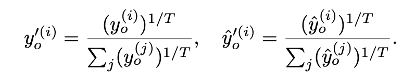The purpose is to increase the weight with less samples.
The following process is represented by a pseudo code algorithm: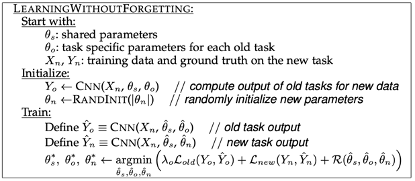The process is very clear. The last line is the key of this article. R refers to some regularization, and the two loss functions have been explained above. Finally, there is one λ Parameter, which determines the importance ratio of new and old tasks in the training process, generally 1, so that both ends can be taken into account.

4.2 MAS

Memory aware synapses: learning what (not) to forget. This article is different from the above two in that it calculates and updates the strength of each parameter. This paper first gives a comparison with the above two methods: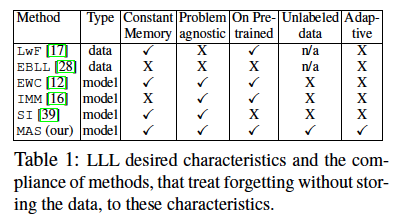The author himself said that in fact, his own method is better everywhere. Low cost, wide field, unsupervised learning can also be used, and reserve capacity for future tasks. Constant memory: whether the memory occupied by the model is a constant, because only a constant can avoid explosion due to the increase of subsequent tasks. Problem agnostic: can the model only solve one problem? The model should be able to perform well and apply to all fields. On trained: given a pre trained model, you can make changes on its top, and then add new tasks. Unlabelled data: can the model be used for unsupervised learning? This is a fatal problem, which determines many directions and whether the model can be learned. Adaptive: whether the model can leave enough space for each task.
The main idea of this paper is to calculate the strength Ω of each parameter, so as to limit the update strength of the parameter according to this strength. Whenever a new task comes in to train it, for the parameter with large Ω, try to reduce its change range in the gradient descent, because this parameter is very important to a task in the past, and its value needs to be retained to avoid catastrophic forgetting. For the parameter with small Ω, we can update it gradient in a large range to obtain better performance or accuracy on the new task. In the specific training process, the intensity Ω is added to the loss function in the form of regular term.

4.2.1 strength calculation

The idea is that if a parameter changes and has a great impact on the model, the strength of this parameter should be great. The authors regard the degree of change of this model as the strength of the parameter.
First, assume that f is an approximate function of the forward propagating real function δ Is a disturbance parameter, then:On the left is the measurement of the change intensity caused by parameter changes, and on the right is the specific practice.
In fact, it is natural to think that the gradient must be used first to measure the intensity of change. Then, the gradient only needs a first-order derivative.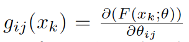And the strength Ω:However, considering the multidimensional situation, it is necessary to calculate for each dimension, which is not in line with the easy style of our computer specialty, so the author uses a square of two normal forms to replace the calculation method of this g function, so that all dimensions can be unified into one dimension, so that all contents can be obtained after one calculation.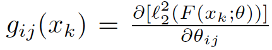Then, how to calculate the loss of the whole model? Back to the familiar loss:
He will constrain the direction of the gradient according to the intensity.5. Summary

In general, we have seen the general methods of continuous learning. Summarize the continuous approach:
1、regularization
2. Training new model, model aggregation
3. Data before repeated training
4. Long and short-term memory, constantly integrating short-term memory into long-term memory
5. As far as possible, let the learned knowledge be recorded on a few neurons
In fact, the feeling is not that each method must be like this, but that specific methods need to be selected according to the actual application to solve specific problems. Each method has its own suitability and application.
Like EWC, this method is very general, but the disadvantage is also obvious, that is, the intensity of the overall change is consistent, so there is no distinction, and the model may not be optimal.
Like LWF, this is very similar to model aggregation. Using knowledge distillation can better retain the previous tasks. And this also applies to model aggregation.
Like MAS, it is more detailed and independent of data. It is also a general algorithm.
The future direction of AI development will also depend on continuous learning rather than offline training algorithms. Human beings learn in this way, and artificial intelligence systems will be more and more capable of doing so. Imagine going to an office for the first time and tripping over an obstacle. The next time you go there, maybe just a few minutes later, you’ll probably know to be careful of tripping objects.
In short, this field is a broad field, and the problems to be solved are also complex and diverse. There are problems that need to protect users’ privacy and can not reuse data, heterogeneous models and so on. In short, we need to see more and understand more, so as to continuously improve. Specific schemes should also be adopted according to practical problems.

6. References

 Kirkpatrick J, Pascanu R, Rabinowitz N, et al. Overcoming catastrophic forgetting in neural networks[J]. Proceedings of the national academy of sciences, 2017, 114(13): 3521-3526.
 Li Z, Hoiem D. Learning without forgetting[J]. IEEE transactions on pattern analysis and machine intelligence, 2017, 40(12): 2935-2947.
 Aljundi R, Babiloni F, Elhoseiny M, et al. Memory aware synapses: Learning what (not) to forget[C]//Proceedings of the European Conference on Computer Vision (ECCV). 2018: 139-154.

Seven solutions for distributed transactions

1、 What is distributed transaction Distributed transaction means that transaction participants, transaction supporting servers, resource servers and transaction managers are located on different nodes of different distributed systems. A large operation is completed by more than n small operations. These small operations are distributed on different services. For these operations, either all of them are […]